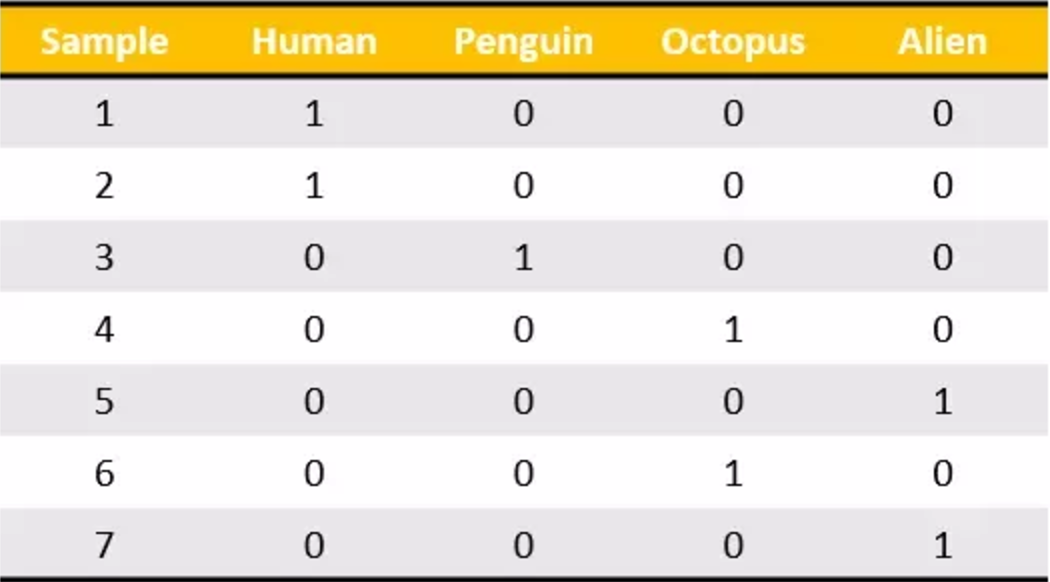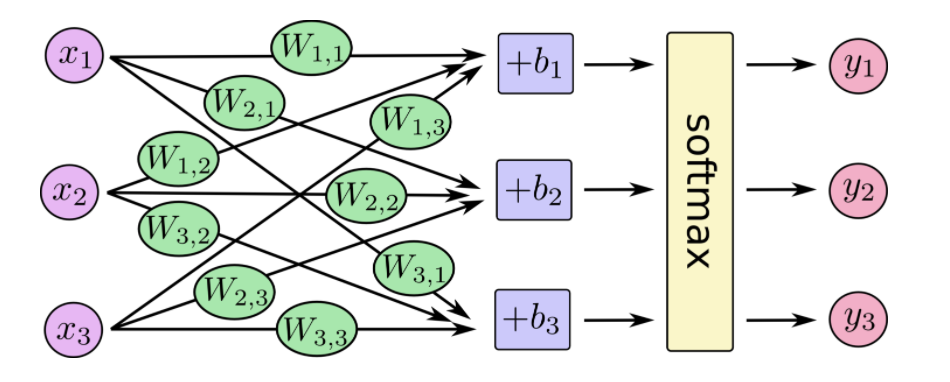# ANN网络分析

## Mnist手写数字识别

Mnist数据集可以从官网下载，网址： http://yann.lecun.com/exdb/mnist/ 下载下来的数据集被分成两部分：55000行的训练数据集（mnist.train）和10000行的测试数据集（mnist.test）。每一个MNIST数据单元有两部分组成：一张包含手写数字的图片和一个对应的标签。我们把这些图片设为“xs”，把这些标签设为“ys”。训练数据集和测试数据集都包含xs和ys，比如训练数据集的图片是 mnist.train.images ，训练数据集的标签是 mnist.train.labels。MNIST中的每个图像都具有相应的标签，0到9之间的数字表示图像中绘制的数字。用的是one-hot编码### one-hot编码tf.one_hot

tf.one_hot(indices, depth, on_value=None, off_value=None, axis=None, dtype=None, name=None)

• indices 在独热编码中位置，即数据集标签
• depth 张量的深度，即类别数
indices = [0, 1, 2, 1]
depth = 3

[[1., 0., 0.],
[0., 1., 0.],
[0., 0., 1.],
[1., 1., 0.]]


### SoftMax回归

MNIST中的每个图像都是零到九之间的手写数字。所以给定的图像只能有十个可能的东西。我们希望能够看到一个图像，并给出它是每个数字的概率。例如，我们的模型可能会看到一个九分之一的图片，80％的人肯定它是一个九，但是给它一个5％的几率是八分之一（因为顶级循环），并有一点概率所有其他，因为它不是100％确定。这是一个经典的情况，其中softmax回归是一种自然简单的模型。如果要将概率分配给几个不同的东西之一的对象，softmax是要做的事情，因为softmax给出了一个[0,1]之间的概率值加起来为1的列表。稍后，当我们训练更复杂型号，最后一步将是一层softmax。

softmax回归有两个步骤：首先我们将我们的输入的证据加在某些类中，然后将该证据转换成概率。每个输出的概率，对应着独热编码中具体的类别。

$\text{softmax}(x)_i=\frac{\exp(x_i)}{\sum_j\exp(x_j)}$$y=\text{softmax}(Wx + b)$

### 损失计算-交叉熵损失

$H_{y'}(y){=}-\sum_iy'_ilog\left(y_i\right)$

tf.nn.softmax_cross_entropy_with_logits

tf.nn.softmax_cross_entropy_with_logits(_sentinel=None, labels=None, logits=None, dim=-1, name=None)

• labels 独热编码过的标签值
• logits 没有log调用过的输入值
• 返回 交叉熵损失列表
tf.nn.softmax_cross_entropy_with_logits(labels=y_label, logits=y))


## 实现神经网络模型

### 获取数据

tensorflow提供给我们下载mnist数据集的接口，需要指定下载目录

from tensorflow.examples.tutorials.mnist import input_data

# 输入数据


### 计算数据

# 建立输入数据占位符
x = tf.placeholder(tf.float32, [None, 784])

#初始化权重和偏置
W = tf.Variable(tf.zeros([784, 10]))
b = tf.Variable(tf.zeros())

# 输出结果
y = tf.matmul(x, W) + b


### 梯度下降优化与训练

train_step = tf.train.GradientDescentOptimizer(0.5).minimize(cross_entropy)


# 训练
for i in range(1000):
print("第%d次训练"%(i))
batch_xs, batch_ys = mnist.train.next_batch(100)
sess.run(train_step, feed_dict={x: batch_xs, y_label: batch_ys})


### 模型正确率评估

correct_prediction = tf.equal(tf.argmax(y, 1), tf.argmax(y_label, 1))


accuracy = tf.reduce_mean(tf.cast(correct_prediction, tf.float32))


### 跟踪变量

tf.summary.scalar("loss",cross_entropy)

tf.summary.scalar("accuracy", accuracy)

tf.summary.histogram("W",W)


## 完整代码：

from __future__ import absolute_import
from tensorflow.examples.tutorials.mnist import input_data

import tensorflow as tf

FLAGS = tf.app.flags.FLAGS

tf.app.flags.DEFINE_string('data_dir', '/tmp/tensorflow/mnist/input_data',
"""数据集目录""")
tf.app.flags.DEFINE_integer('max_steps', 2000,
"""训练次数""")
tf.app.flags.DEFINE_string('summary_dir', '/tmp/summary/mnist/train',
"""事件文件目录""")

def main(sess):
# 输入数据

# 建立输入数据占位符
x = tf.placeholder(tf.float32, [None, 784])

# 初始化权重和偏置
W = tf.Variable(tf.zeros([784, 10]))
b = tf.Variable(tf.zeros())

# 输出结果y
y = tf.matmul(x, W) + b

# 建立类别占位符
y_label = tf.placeholder(tf.float32, [None, 10])

# 计算交叉熵损失平均值
cross_entropy = tf.reduce_mean(tf.nn.softmax_cross_entropy_with_logits(labels=y_label, logits=y))
# 生成优化损失操作
# 比较结果
correct_prediction = tf.equal(tf.argmax(y, 1), tf.argmax(y_label, 1))
# 计算正确率平均值
accuracy = tf.reduce_mean(tf.cast(correct_prediction, tf.float32))

tf.summary.scalar("loss",cross_entropy)

tf.summary.scalar("accuracy", accuracy)

tf.summary.histogram("W",W)

tf.global_variables_initializer().run()

# 合并所有摘要
merged = tf.summary.merge_all()
summary_writer = tf.summary.FileWriter(FLAGS.summary_dir, graph=sess.graph)

# 训练
for i in range(1000):

print("第%d次训练"%(i))
batch_xs, batch_ys = mnist.train.next_batch(100)

sess.run(train_step, feed_dict={x: batch_xs, y_label: batch_ys})

print(sess.run(accuracy,feed_dict={x: batch_xs, y_label: batch_ys}))

summary = sess.run(merged,feed_dict={x: batch_xs, y_label: batch_ys})